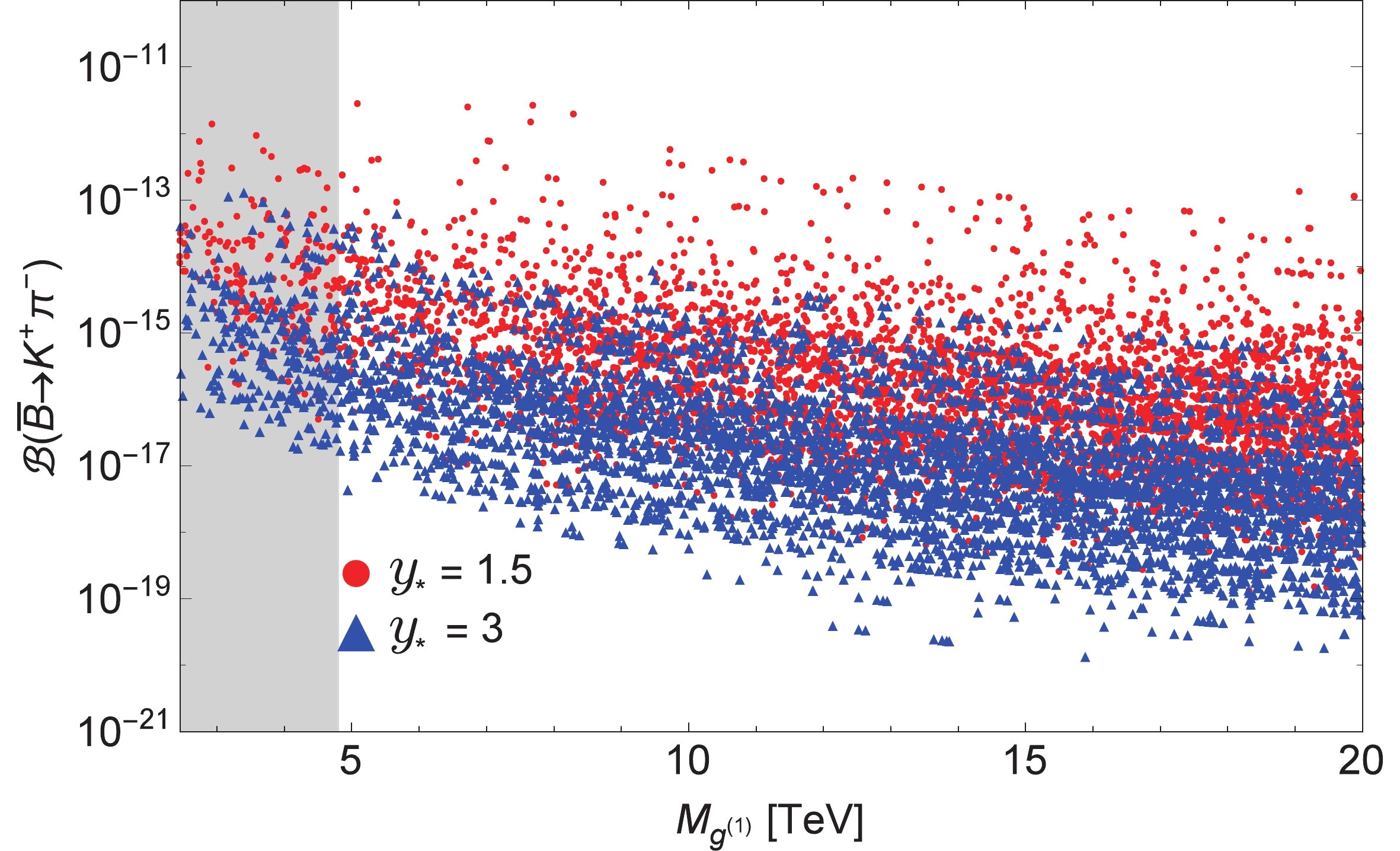# New physics search in the doubly weak decay ${{\overline B}^0 \to K^+ \pi^- }$• The doubly weak transition $b\to dd{\bar s}$is highly suppressed in the Standard Model, which makes it a potential channel for exploring new physics signals. We present a study of the exclusive two-body wrong sign weak decay $\smash{\overline B}^0\to K^+\pi^-$, which belongs to this class, in the perturbative QCD framework. We perform a model independent analysis for various effective dimension-6 operators for which large effects are possible. We further analyze the considered process in the Randall-Sundrum model, including the custodially protected and the bulk-Higgs Randall-Sundrum models. Exploring the experimentally favored parameter space of these models leads to a large and significant enhancement of the decay rate compared to the Standard Model, which might be accessible in future experiments. We propose to search for the wrong sign decay $\smash{\overline B}^0\to K^+\pi^-$via flavor-tagged time-dependent analyses, which can be performed at LHCb and Belle-II.
••Get Citation
Faisal Munir Bhutta, Ying Li, Cai-Dian Lü and Yue-Hong Xie. New physics search in the doubly weak decay ${{\overline B}^0 \to K^+ \pi^- }$[J]. Chinese Physics C, 2019, 43(7): 073102. doi: 10.1088/1674-1137/43/7/073102
Faisal Munir Bhutta, Ying Li, Cai-Dian Lü and Yue-Hong Xie. New physics search in the doubly weak decay ${{\overline B}^0 \to K^+ \pi^- }$[J]. Chinese Physics C, 2019, 43(7): 073102.Milestone
Revised: 2019-04-11
Article Metric

Article Views(852)
Cited by(0)
Policy on re-use
To reuse of Open Access content published by CPC, for content published under the terms of the Creative Commons Attribution 3.0 license (“CC CY”), the users don’t need to request permission to copy, distribute and display the final published version of the article and to create derivative works, subject to appropriate attribution.
###### 通讯作者: 陈斌, bchen63@163.com
• 1.

沈阳化工大学材料科学与工程学院 沈阳 110142

Title:
Email:

## New physics search in the doubly weak decay ${{\overline B}^0 \to K^+ \pi^- }$###### Corresponding author: Yue-Hong Xie, yuehong.xie@cern.ch
• 1. Institute of High Energy Physics, Chinese Academy of Sciences, Beijing 100049, China
• 2. Department of Physics, Yantai University, Yantai 264005, China
• 3. University of Chinese Academy of Sciences, Beijing 100049, China
• 4. Institute of Particle Physics, Central China Normal University, Wuhan 430079, China
• 5. Institute of Theoretical Physics, College of Applied Sciences, Beijing University of Technology, Beijing 100124, China

Abstract: The doubly weak transition $b\to dd{\bar s}$is highly suppressed in the Standard Model, which makes it a potential channel for exploring new physics signals. We present a study of the exclusive two-body wrong sign weak decay $\smash{\overline B}^0\to K^+\pi^-$, which belongs to this class, in the perturbative QCD framework. We perform a model independent analysis for various effective dimension-6 operators for which large effects are possible. We further analyze the considered process in the Randall-Sundrum model, including the custodially protected and the bulk-Higgs Randall-Sundrum models. Exploring the experimentally favored parameter space of these models leads to a large and significant enhancement of the decay rate compared to the Standard Model, which might be accessible in future experiments. We propose to search for the wrong sign decay $\smash{\overline B}^0\to K^+\pi^-$via flavor-tagged time-dependent analyses, which can be performed at LHCb and Belle-II.

### HTML2.   ${{\overline B}^0 \to K^+ \pi^-}$ decay in the Standard Model3.   Model independent analysis of the ${{\overline B}^0\to K^+\pi^-}$ decay4.   ${{\overline B}^0 \to K^+ \pi^-}$ in the custodial RS model5.   ${{\overline B}^0\to K^+\pi^-}$ in the bulk-Higgs RS model6.   Numerical results for the RS modelsA.   Appendix A: Wave functionsB.   Appendix B: Analytic formulae
Reference (55)
PDF查看关注分享

Top

### 目录/DownLoad:  Full-Size Img  PowerPoint